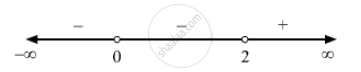Advertisement Remove all ads

# Write the Solution of the Inequation X 2 X − 2 > 0 - Mathematics

Write the solution of the inequation$\frac{x^2}{x - 2} > 0$

Advertisement Remove all ads

#### Solution

$\text{ We have },$
$\frac{x^2}{x - 2} > 0$
$\text{ Equating both the numerator and the denominator with zero, we obtain x = 0 and x = 2 as critical points } .$
$\text{ Plotting these points on the real line, we see that the real line is divided into three regions } .\[\text{ Therefore, the solution set of the given inequality is } x \in (2, \infty )$

Is there an error in this question or solution?
Advertisement Remove all ads

#### APPEARS IN

RD Sharma Class 11 Mathematics Textbook
Chapter 15 Linear Inequations
Q 1 | Page 32
Advertisement Remove all ads

#### Video TutorialsVIEW ALL 

Advertisement Remove all ads
Share
Notifications

View all notifications

Forgot password?